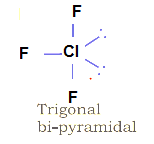# VSEPR Theory (Valence Shell Electron Pair Repulsion Theory)

## VSEPR Theory (Valence Shell Electron Pair Repulsion Theory)

It is given by Sidgwick and Powell. This theory is based on repulsive character of electron pairs in the valence shell of atoms
Main postulates are
i) The exact shape of molecule depends upon no of electron pairs (bonder or non – bonded) around the central atom.
ii) The electron pairs tend to repel each other since they exist around the central atom and the e- clouds are negatively charged.
iii) electron pairs try to take such position which can minimize the repulsion b/w them.
iv) The valence shell is taken as a sphere with the electron pairs placed at distance.
v) A multiple bond is treated as if it is a single electron pair & the electron pairs which constitute the bond as a single pair.
vi) The maximum repulsion is between lone pair electron and lone pair electron and min. between bond pair electron and bond pair electron
vii) The bond pairs and lone pairs minimize the repulsion and take the position with minimum repulsion.
viii) The repulsive interaction of electron pairs decreases in the order:
Lone pair (lp) – Lone pair (lp) > Lone pair (lp) – Bond pair (bp) > Bond pair (bp) – Bond pair (bp)

## How to Predict the shape of the Molecule based on it

we can predict the shape of the molecules by dividing the molecules in two catagories
(i) molecules in which the central atom has no lone pair
(ii) molecules in which the central atom has one or more lone pairs.
Case -1 molecules in which the central atom has no lone pair
In these molecules, the central atom does not have any lone pair and it just have bonded pair
A) Molecules where central atom have two bonded pair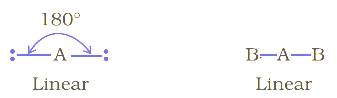Molecular geometry : Linear
Examples BeCl2
B) Molecules where central atom have three bonded pair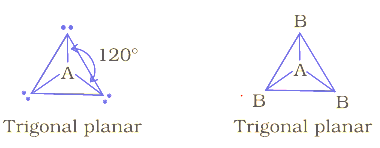Molecular geometry : Trigonal  Planar
Examples : BF3
C) Molcules where central atom have four bonded pair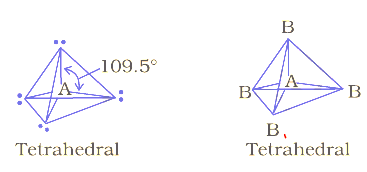Here in this  central atom is located at the center with four substituents that are located at the corners of a tetrahedron.
Molecular geometry : tetrahedron
Examples  CH4
D) Molecules where central atom has five bonded pair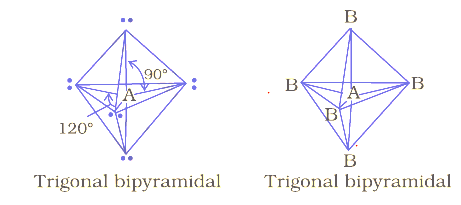Here Three of the bonds are arranged along the central atom’s equator, with 120° angles between them; the other two are placed at the atom’s axis. Axial bonds are at right angles to the equatorial bonds
Molecular geometry : Trigonal bipyramidal
Examples  PCl5
Case -2 molecules in which the central atom has one or more lone pair
A) Lone Pair -1  and Bond Pair 2
Electron Geometry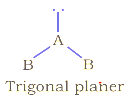Shape Of molecule : Bent
Example : SO2 ,O3
B) Lone Pair -1  and Bond Pair 3
Electron Geometry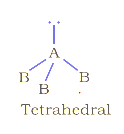Shape Of molecule : Trigonal pyramid. Angle between should be 109.50 but it is reduced to 1070  as the lp-bp repulsion is more than bp-bp repulsion
Example : NH3
C) Lone Pair -2  and Bond Pair 2
electron Geometry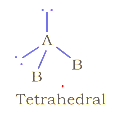Shape : Bent. Theoretically, Angle between should be 109.50 but it is reduced to 104.50  as lp-lp repulsion is more than lp-bp repulsion which is more
than bp-bp repulsion.
Example : H2 O
D) Lone Pair-1 , Bond Pair -4
electron Geometry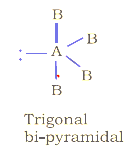Shape: distorted tetrahedron, a folded square or a see-saw.
Example :SF4

## Solved Examples

Question
Give reason why Ammonia is pyramidal and water is bend
In NH3 due to bp – lp repulsion which is more than bp – bp repulsion, the bond angle reduces to 107o instead of 109.5o
In water due to two lone pairs, lp – lp repulsion ones are much more than bp – bp repulsion. The bond angle reduces to 104.5o instead of 109.5o
Question
S F4 is sea – saw in shape. Why?
When lp is present at exit position there are more bp – lp repulsion while if lp is present at equatorial position there are less bp – lp repulsion. Therefore, later one is more stable condition. Hence, S F4 has sea – saw shape.
Question
Cl F3 is T – shaped. Why?
Lps when present at equatorial positions there are least lp – bp repulsion therefore it is having T – shaped
Due to lp – bp repulsion it occupies bent T – shape.